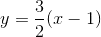# Limit as x approaches infinity

SprucerMoose
Gday,
I was contemplating limits today and tried to prove something to myself and I can't figure it out.

This is what I am trying to wrap my head around.

http://latex.codecogs.com/gif.latex?\lim_{x \to \infty } \sqrt{x^2 + 1} [Broken]

I'm not looking for the "numerical" solution of infinity, what I am trying to prove is that as x approaches infinity, this expression approaches x.

I then came to this conclusion.

http://latex.codecogs.com/gif.latex?\lim_{x \to \infty } \sqrt{x^2 + 1} = \lim_{x \to \infty } \sqrt{x^2(1 + \frac{1}{x^2})} = \lim_{x \to \infty } x\sqrt{1 + \frac{1}{x^2}} = x\sqrt{1 + 0} = x [Broken]

Is this logic correct? I can't see why it wouldn't be. But this way of thinking leads to a scenario that I am not happy with.

http://latex.codecogs.com/gif.latex?\lim_{x \to \infty } \sqrt{(x-1)^2 + 1} = \lim_{x \to \infty } \sqrt{x^2 - 2x +2} = \lim_{x \to \infty } \sqrt{x^2( 1 - \frac{2}{x} +\frac{2}{x^2})} = \lim_{x \to \infty} x\sqrt{1 - \frac{2}{x} + \frac{2}{x^2}} = x\sqrt{1 - 0 + 0} = x [Broken]

I am reasonably certain that the solution to this particular expression should be http://latex.codecogs.com/gif.latex?\lim_{x \to \infty } \sqrt{(x-1)^2 + 1} = x - 1 [Broken].

Can someone please tell me where I have gone wrong?

Last edited by a moderator:

## Answers and Replies

Staff Emeritus
Homework Helper
$$\lim_{x \to \infty } x\sqrt{1 + \frac{1}{x^2}} = x\sqrt{1 + 0}$$

This is where you have gone wrong. x is a variable in the limit. And when you calculate the limit, there is no way that there could be an x in the solution.

SprucerMoose
Thanks for the response.

So how would I prove the existance of the asymptotes of a hyperbola for example, or other oblique asymptotes for that matter?

Thanks for the response.

So how would I prove the existance of the asymptotes of a hyperbola for example, or other oblique asymptotes for that matter?

Same method - evaluating the limits - except you will get a constant based on the properties of the hyperbola, not on x.

SprucerMoose
I'm still stuck.

http://latex.codecogs.com/gif.latex?\frac{(x-1)^2}{4} - \frac{y^2}{9} = 1 [Broken]

http://latex.codecogs.com/gif.latex?y = \pm \sqrt{\frac{9(x-1)^2}{4} -9} [Broken]

http://latex.codecogs.com/gif.latex?\lim_{x \to \infty} \sqrt{\frac{9(x-1)^2}{4} -9} [Broken]

http://latex.codecogs.com/gif.latex?\lim_{x \to \infty} \frac{3}{2}\sqrt{(x-1)^2 -4} [Broken]

What would I need to do to prove this expression approachesas x approaches infinity?

Last edited by a moderator:
Homework Helper
Here's what I would do.
$$\frac{(x- 1)^2}{4}- \frac{y^2}{9}= 1$$
$$\left(\frac{x-1}{2}+ \frac{y}{3}\right)\left(\frac{x-1}{2}- \frac{y}{3}\right)= 1$$

For x and y both very large positive numbers,
$$\frac{x-1}{2}+ \frac{y}{3}$$
will be a very large postive number.

For x and y both very large negative numbers,
$$\frac{x-1}{2}+ \frac{y}{3}$$
will be a very large negative number.

In either case, we can divide both sides by it and get
$$\frac{x-1}{2}- \frac{y}{3}= \frac{1}{\frac{x-1}{2}+ \frac{y}{3}}$$
so that
$$\frac{x-1}{2}- \frac{y}{3}\approx 0$$
so that
$$\frac{x-1}{2}\approx \frac{y}{3}$$

If x and y are large but of different signs, then
$$\frac{x-1}{2}- \frac{y}{3}$$
will be a large positive number (if x is positive and y is negative) or a large negative number (if x is negative and y is positive). In either case, we can divide both sides of the original equation by
$$\frac{x-1}{2}- \frac{y}{3}$$
to get that
$$\frac{x-1}{2}\approx -\frac{y}{3}$$

Last edited by a moderator:
This seems to be confusing to me; the limit itself approaches infinity; you are looking for asymptotes. I mean, as x approaches infinity, so does the function. Its asymptote, though, it that equation you posted. I think it's abuse of notation, somehow, to find the function of the asymptote while using x as the variable in the limit? (it causes this confusion).

SprucerMoose
HallsofIvy, thanks so much. That's heaps better. But I'm still wondering what the process is to find the function that an expression approaches while tending to infinity and is under a square root. I have no problem calculating oblique asymptotes when i can use polynomial long devision, but cannot find a way to calculate the limits I originaly posted.

Please tell me there is a method.

 I guess I'm not looking for the actual limit, but rather the function of the oblique asymptote. Poor notation on my part. How would I set up the original (not the hyperbola) problem with correct notation?

Last edited:
TylerH
This is where you have gone wrong. x is a variable in the limit. And when you calculate the limit, there is no way that there could be an x in the solution.

Why not show the correct way, as opposed to just saying it is wrong?

The problem, as micromass said, is that x is the variable of the limit, and is only defined within the limit. You must say $$\lim_{x \to \infty } x\sqrt{1 + \frac{1}{x^2}} = \lim_{x \to \infty } x\sqrt{1 + 0}$$ and you can only remove the $$\lim_{x \to \infty }$$ when you have either found the limit, in terms other than x, or you've found that there is not limit.

As for why it doesn't work if you expand the binomial, I don't know. But, the way you can get the right answer is to $$\lim_{x \to \infty } \sqrt{\left( x-1 \right)^2 +1} = \lim_{x \to \infty } \sqrt{\left( x - 1 \right)^2 \left(1 + \frac{1}{\left( x - 1 \right)^2} \right) }$$ and continue the obvious.